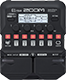# creedence

Discussion in 'Zoom G1/G1X Four' started by joaoliria, Sep 8, 2022.

1. creedenceDevice: Zoom G1 Four
Firmware: 2.00

Name on device: Violao 2
Optimized for: Phones/Speaker

Effects chain:ccr

Effect: "ZNR" (Dynamics), active - "yes"
"Detect" = GTRIN
"Depth" = 100
"Threshold" = 40
"Decay" = 0

Effect: "Aco.Sim" (Overdrive / Distortion), active - "yes"
"Top" = 65
"Body" = 77
"Tone" = 90
"Volume" = 90

Effect: "RackComp" (Dynamics), active - "yes"
"Threshold" = 0
"Ratio" = 5
"Attack" = 7
"Volume" = 80

Effect: "Gt GEQ 7" (Filter), active - "yes"
"100" = 0.0
"200" = 0.0
"400" = 0.0
"800" = 0.0
"1.6k" = 0.0
"3.2k" = 0.0
"6.4k" = 0.0
"VOL" = 100

Effect: "Hall" (Reverb), active - "yes"
"PreD" = 1
"Decay" = 3
"Mix" = 37
"Tail" = On

Patch Volume: 100